Courses

# The Three Moment Equations-II Civil Engineering (CE) Notes | EduRev

## Civil Engineering (CE) : The Three Moment Equations-II Civil Engineering (CE) Notes | EduRev

The document The Three Moment Equations-II Civil Engineering (CE) Notes | EduRev is a part of the Civil Engineering (CE) Course Structural Analysis.
All you need of Civil Engineering (CE) at this link: Civil Engineering (CE)

Instructional Objectives

After reading this chapter the student will be able to

1. Derive three-moment equations for a continuous beam with yielding supports.
2. Write compatibility equations of a continuous beam in terms of three moments.
3. Compute reactions in statically indeterminate beams using three-moment equations.
4. Analyse continuous beams having different moments of inertia in different spans and undergoing support settlements using three-moment equations.

Introduction

In the last lesson, three-moment equations were developed for continuous beams with unyielding supports. As discussed earlier, the support may settle by unequal amount during the lifetime of the structure. Such future unequal settlement induces extra stresses in statically indeterminate beams. Hence, one needs to consider these settlements in the analysis. The three-moment equations developed in the pervious lesson could be easily extended to account for the support yielding. In the next section three-moment equations are derived considering the support settlements. In the end, few problems are solved to illustrate the method.

Derivation of Three-Moment Equation

Consider a two span of a continuous beam loaded as shown in Fig.13.1. Let ML, MC and MR be the support moments at left, center and right supports respectively. As stated in the previous lesson, the moments are taken to be positive when they cause tension at the bottom fibers. IL and IR denote moment of inertia of left and right span respectively and lL and lR denote left and right spans respectively. Let δLC and δR be the support settlements of left, centre and right supports respectively. δLC and δR are taken as negative if the settlement is downwards. The tangent to the elastic curve at support C makes an angle θCL at left support and θCR at the right support as shown in Fig. 13.1. From the figure it is observed that,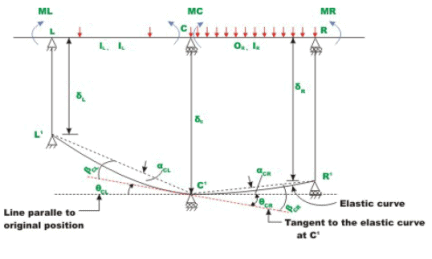Fig. 13.1 Continuous beam with support settlement

θCL = θCR                              (13.1)

The rotations βCL and βCR due to external loads and support moments are calculated from the M/EI diagram .They are (see lesson 12)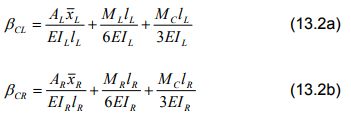The rotations of the chord L' C'' and C'' R' from the original position is given by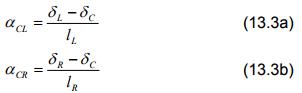From Fig. 13.1, one could write,

θCL = αCL - βCL                          (13.4a)

θCR = βCR - αCR                      (13.4b)

Thus, from equations (13.1) and (13.4), one could write,

αCL − βCL = βCR −αCR                (13.5)

Substituting the values of αCL, αCR, βCL and βCR in the above equation,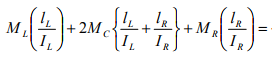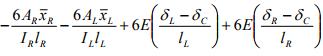This may be written as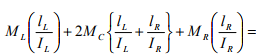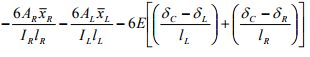(13.6)

The above equation relates the redundant support moments at three successive spans with the applied loading on the adjacent spans and the support settlements.

Example 13.1
Draw the bending moment diagram of a continuous beam BC shown in Fig.13.2a by three moment equations. The support B settles by 5mm below A and C . Also evaluate reactions at A , B and C .Assume EI to be constant for all members and E = 200 GPa , I = 8x106 mm4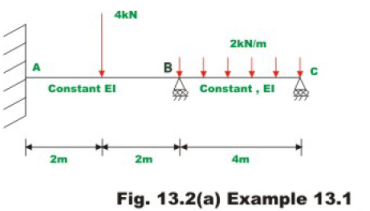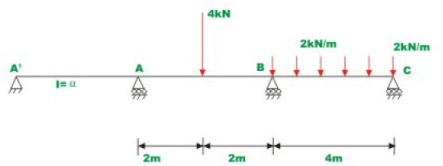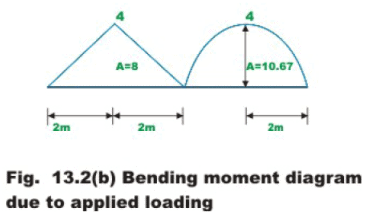Assume an imaginary span having infinitely large moment of inertia and arbitrary span L′ left of A as shown in Fig.13.2b .Also it is observed that moment at C is zero.

The given problem is statically indeterminate to the second degree. The moments MA and MB, the redundants need to be evaluated. Applying three moment equation to the span A’AB,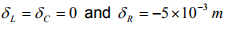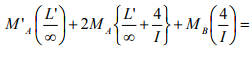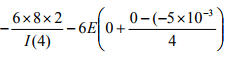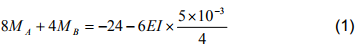Note that,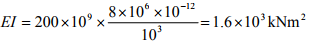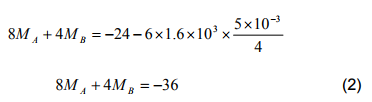Again applying three moment equation to span ABC the other equations is obtained. For this case,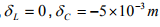(negative as the settlement is  downwards) and δR = 0.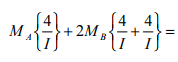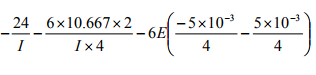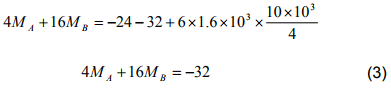Solving equations (2) and (3),

MB = −1.0 kN.m
MA = −4.0 kN.m                          (4)

Now, reactions are calculated from equations of static equilibrium (see Fig.13.2c)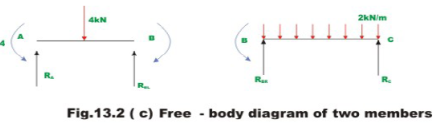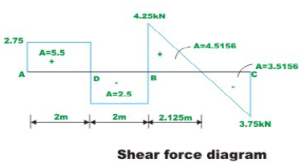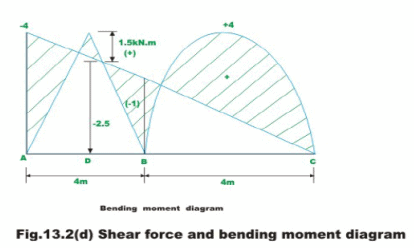Thus,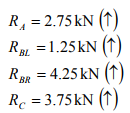The reactions at B,

RB = RBR + RBL = 5.5kN                                  (5)

The area of each segment of the shear force diagram for the given continuous beam is also indicated in the above diagram. This could be used to verify the previously computed moments. For example, the area of the shear force diagram between A and B is 5.5 kN.m. This must be equal to the change in the bending moment between A and D, which is indeed the case (−4-1.5 = 5.5 kN.m). Thus, moments previously calculated are correct.

Example 13.2

A continuous beam ABCD is supported on springs at supports B and C as shown in Fig.13.3a. The loading is also shown in the figure. The stiffness of springs is kB = EI/20 and kC = EI/30. Evaluate support reactions and draw bending moment diagram. Assume EI to be constant.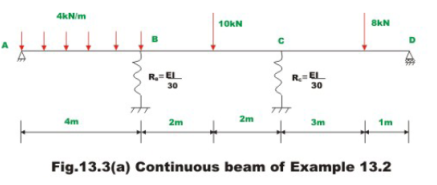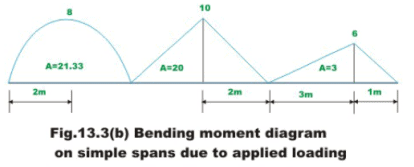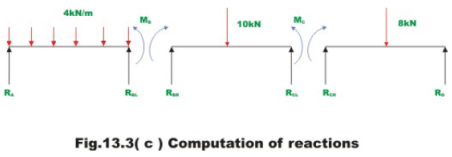In the given problem it is required to evaluate bending moments at supports B and C. By inspection it is observed that the support moments at A and D are zero. Since the continuous beam is supported on springs at B and C, the support settles. Let RB and RC be the reactions at B and C respectively. Then the support settlement at B and C are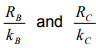respectively. Both the settlements are negative and in other words they move downwards. Thus,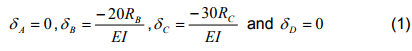Now applying three moment equations to span ABC(see Fig.13.2b)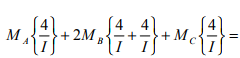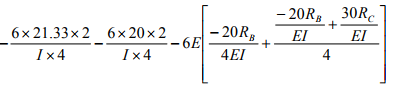Simplifying,

16MB + 4MC = -124 + 60RB - 45RC                             (2)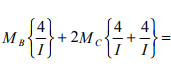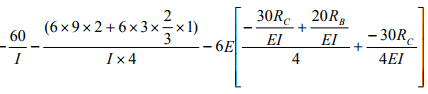4MB + 16MC = -90 + 90RC - 30RB                   (3)

In equation (2) and (3) express RB and RC in terms of MB and MC (see Fig.13.2c)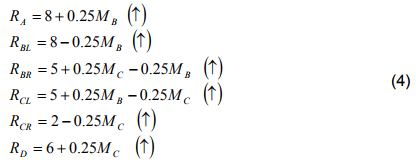Note that initially all reactions are assumed to act in the positive direction (i.e. upwards) .Now,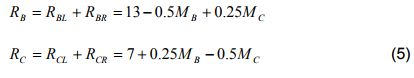Now substituting the values of RB and RC in equations (2) and (3),

16MB + 4MC =-124 + 60(13 - 0.5MB + 0.25MC)- 45(7 + 0.25MB - 0.5MC)

Or,

57.25MB - 33.5MC = 341                                        (6)

Ana from equation 3,

4MB + 16MC = -90 + 90(7 + 0.25MB - 0.5MC)-30(13 - 0.5MB + 0.25MC)

Simplifying,

- 33.5MB + 68.5MC = 150                                       (7)

Solving equations (6) and (7)

MC = 7.147 kN.m

MB = 10.138 kN.m                   (8)

Substituting the values of MB and MC in (4),reactions are obtained.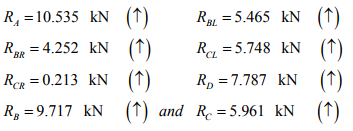The shear force and bending moment diagram are shown in Fig. 13.2d.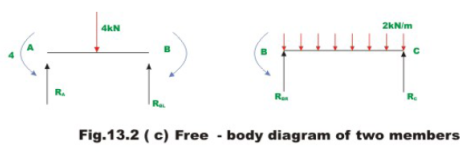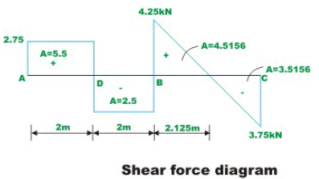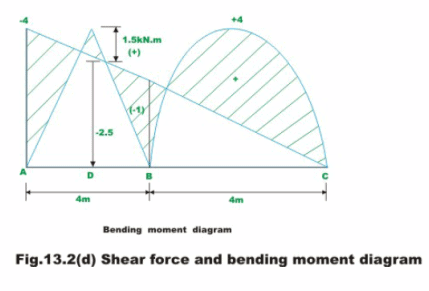Example 13.3
Sketch the deflected shape of the continuous beam ABC of example 13.1. The redundant moments MA and MB for this problem have already been computed in problem 13.1.They are,

MB =-1.0 kN.m

MA = -4.0 kN.m

The computed reactions are also shown in Fig.13.2c.Now to sketch the deformed shape of the beam it is required to compute rotations at B and C. These joints rotations are computed from equations (13.2) and (13.3).
For calculating θA, consider span A’AB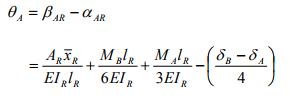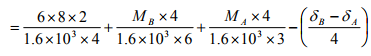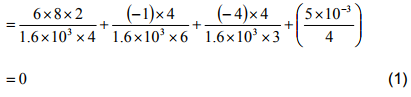For calculating θBL , consider span ABC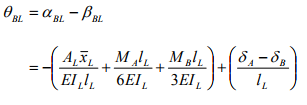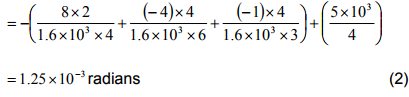For θBR consider span ABC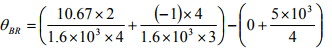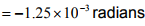(3)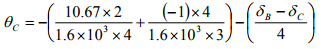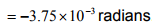(4)

The deflected shape of the beam is shown in Fig. 13.4.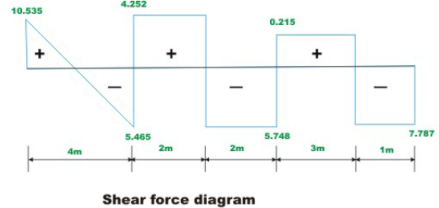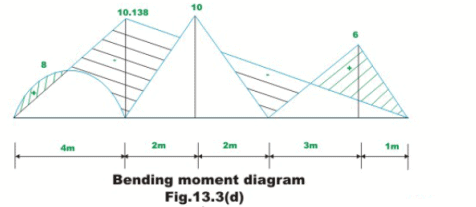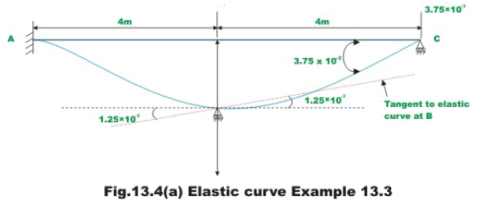Summary

The continuous beams with unyielding supports are analysed using threemoment equations in the last lesson. In this lesson, the three-moment-equations developed in the previous lesson are extended to account for the support settlements. The three-moment equations are derived for the case of a continuous beam having different moment of inertia in different spans. Few examples are derived to illustrate the procedure of analysing continuous beams undergoing support settlements using three-moment equations.

Offer running on EduRev: Apply code STAYHOME200 to get INR 200 off on our premium plan EduRev Infinity!

## Structural Analysis

30 videos|122 docs|28 tests

,

,

,

,

,

,

,

,

,

,

,

,

,

,

,

,

,

,

,

,

,

;